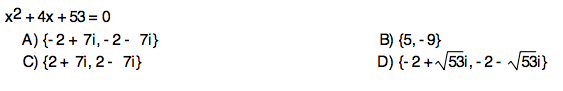starstarstarstarstarstarstarstarstarstar
4 (2 ratings)
by Robert Pieper
| 4 Questions
Note from the author:1
1 pt
Solve the equation above.
A
B
C
D2
1 pt
Solve the equation above.
A
B
C
D3
1 pt
Solve the equation above.
A
B
C
D4
1 pt
Use the discriminant to determine what types of solutions are in the equation above.
A
B
C
D